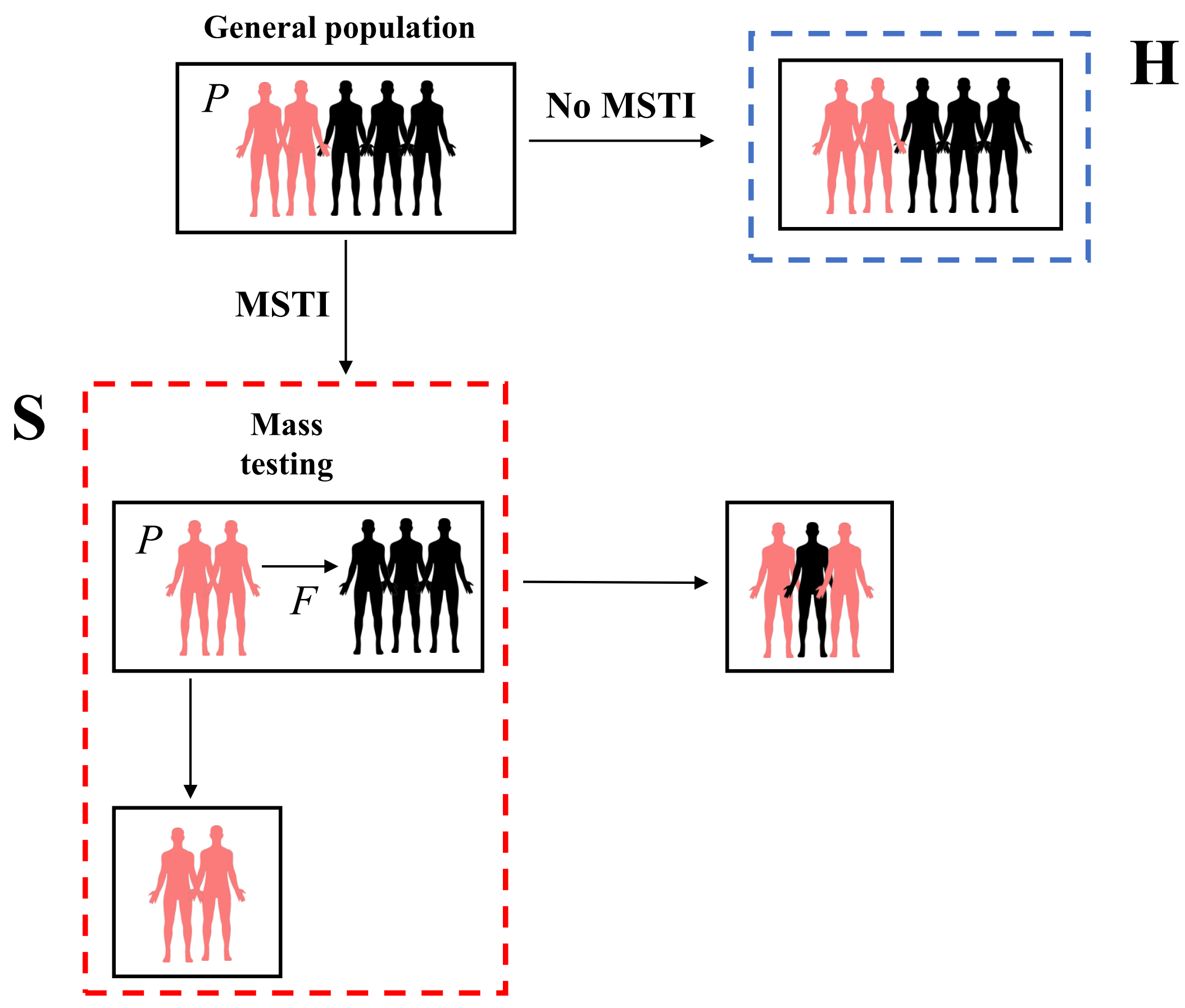A symptom-based mass screening and testing intervention (MSTI) can identify a large fraction of infected individuals during an infectious disease outbreak. China is currently using this strategy for the COVID-19 outbreak. However, MSTI might lead to increased transmission if not properly implemented. We investigate under which conditions MSTI is beneficial.

We consider a scenario where a novel pathogen has entered a population and causes symptoms that are non-specific and similar to circulating pathogens, e.g., general respiratory symptoms. The default is to ask individuals with symptoms to stay home and follow general precautionary measures. An alternative option is to implement MSTI, which requires testing anyone with symptoms specific for the novel pathogen at a healthcare facility.

At any given time during an outbreak, a proportion, \(P\), of those showing symptoms will be infected with the novel pathogen. For symptomatic patients who stay home, the expected number of transmissions of the novel pathogen caused by that person is the average number of transmissions caused by someone who is infected with that pathogen (reproductive number, R), multiplied by the probability the person is infected with the new pathogen, \(T_H = RP\). Similarly, such a patient has a mortality risk due to the novel pathogen of \(M_H = M P\), where \(M\) is the probability of death if a person enters a healthcare facility under regular circumstances, i.e., when they feel bad enough to seek medical care.

For individuals who go through an MSTI, there are two possible scenarios (Figure 1). If the symptomatic individual is infected with the novel pathogen (probability \(P\)), we assume that they are correctly identified and placed under isolation and treatment, where they have reduced transmission and mortality risks, namely \(R_I P\) and \(M_I P\). Importantly, if the health system is strained, those patients arriving at the testing facility without being infected with the novel pathogen (probability \(1-P\)) may become infected by another person in the testing facility. We denote this probability of becoming infected with \(F\). Since these individuals test negative, we assume they will be sent home and then have the same transmission and mortality risk as individuals asked to remain at home. Thus, the total transmission potential for a person undergoing screening is \(T_S = R_I P + R F (1-P)\) and the total mortality risk is \(M_S = M_I P + M F (1-P)\).Figure 1: Structure of the model

We can define transmission and mortality risk ratios (TRR and MRR), which are given by \(TRR=T_S/T_H = R_I / R + (1-P) F/P\) and \(MRR=M_S/M_H= M_I / M_0 + (1-P) F/P\). If that expression is less than 1, it suggests that MSTI reduces transmission and mortality. If it is larger than 1, the better strategy would be to not implement MSTI.

As a best-case scenario, we consider a situation where isolation and treatment following a positive test are perfect, with \(R_I = M_I = 0\) (other scenarios are explored in the SM). In that case, TRR and MRR are the same and given by \((1-P)F/P\). To keep this ratio below 1, the risk of MSTI associated infection needs to be \(F<P/(1-P)\).

Figure 2 shows a heatmap for TRR or MRR (on a log scale) for different values of \(F\) and \(P\). The solid line is given by \(F=P/(1-P)\), at which TRR/MRR=1 (thus their log is 0). This line marks the threshold at which MSTI changes from being beneficial to not being beneficial.

For small \(P\), this is essentially a linear relationship, \(F \approx P\). This means that if the fraction of those infected with the novel pathogen among those with symptoms is low, MSTI is only suitable if the risk of infection associated with MSTI is less than the fraction of symptomatic who carry the novel pathogen. \(P\) will be low if the overall pathogen prevalence is low (e.g. early in an outbreak) and if symptoms are so broad that only a small fraction of those individuals with symptoms are infected with the novel pathogen. As \(P\) increases, a larger MSTI associated infection risk is acceptable. Once \(P\) reaches a value of \(\ge 0.5\), MSTI becomes useful even if \(F=1\), i.e. MSTI associated infection is certain. If such a high \(P\) is reached because the novel pathogen is widespread, MSTI is likely logistically unfeasible. If instead a high \(P\) can be reached by better discrimination of symptoms, MSTI can be a useful strategy.